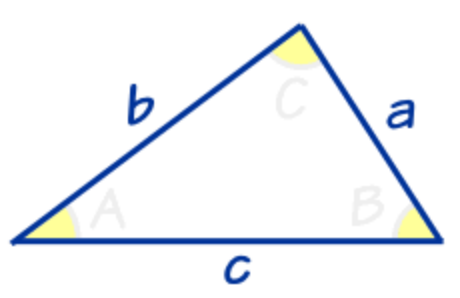# Question

If the ratio of 3 sides of a triangle XYZ is x : y : z = 4 : 8 : 5, can we find the angles of triangle XYZ? If yes, what is the largest angle?

3 Answers

# Answer

Well, that’s great that you find the answer to your question. If you face any other problems regarding this please let me know. But for now,  My sister asks me to find the best essay writing service for her. But I don’t know how to find it so If anyone here knows regarding this please let me know.

0 Replies 0 Likes

These formulas bring back the old school day memories …..

You’re helpful ! guys.

0 Replies 0 Likes

Thank you, Ender. Very useful link.

0 Replies 0 LikesHere’s a good article that explains how to use the law of cosines to find angles in a triangle when all 3 sides are given.

The largest angle C will be the angle formed from the 2 sides a=4u and b=5u.  The longest side c=8u will form the base of the triangle.

Hence, cos (C) = (a2 + b2 – c2) / 2ab = (42 + 52 – 82) / 2(4)(5) = -0.575

Hence C = cos-1(-0.575) = 125.1o

#Corrected 9 Oct.

1 Reply 0 LikesHere’s a good article that explains how to use the law of cosines to find angles in a triangle when all 3 sides are given.

The largest angle C will be the angle formed from the 2 sides a=4u and b=5u.  The longest side c=8u will form the base of the triangle.

Hence, cos (C) = (a2 + b2 – c2) / 2ab = (42 + 52 – 82) / 2(4)(5) = -0.575

Hence C = cos-1(-0.575) = 125.1o

#Corrected 9 Oct.

1 Reply 0 Likes

Thanks, Chief. You have been a great help. But I believe you made a computational error. Should be -0.575

🙂

1 Reply 0 Likes